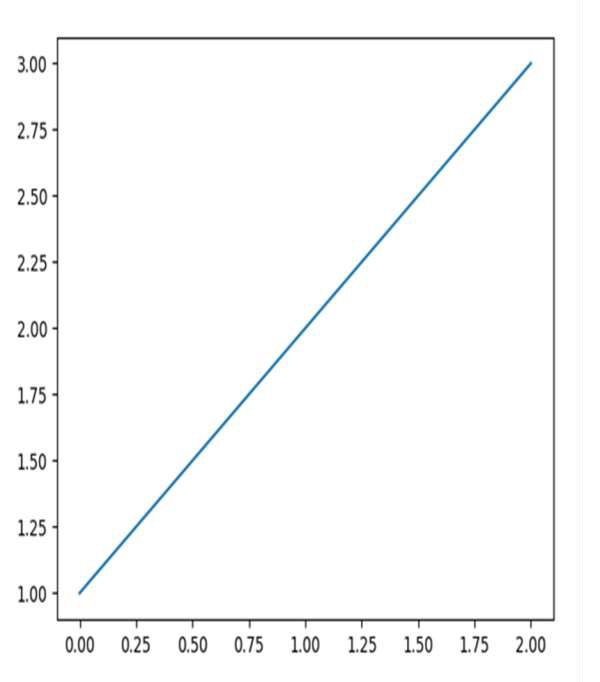# How to save a figure remotely with pylab in Python?

Using the savefig method of the pyplot package, we can save the figure remotely by specifying the location of the figure.

## Steps

• To use a different backend, set it using matplotlib.use('Agg') method.

• Plot the lines using plot() method.

• Using savefig() method, we can save the image remotely, just putting the directory.

• To show the figure, use plt.show().

## Example

import matplotlib

matplotlib.use('Agg')
from matplotlib import pyplot as plt

plt.plot([1, 2, 3])
plt.savefig("remotely_fig.png")

## OutputUpdated on: 17-Mar-2021

306 Views